Computer Science

# Practice Midterm

Try taking this practice test without looking at the answers. These questions are similar to what will be on the midterm.

## Bit

### Question 1

What image will the following code draw?

from byubit import Bit

def turn(bit):
bit.left()
bit.move()
bit.left()

@Bit.run_from_empty(5, 3)
def run(bit):
while bit.front_clear():
bit.move()
bit.paint('green')
turn(bit)
while bit.front_clear():
bit.move()
bit.paint('blue')

a.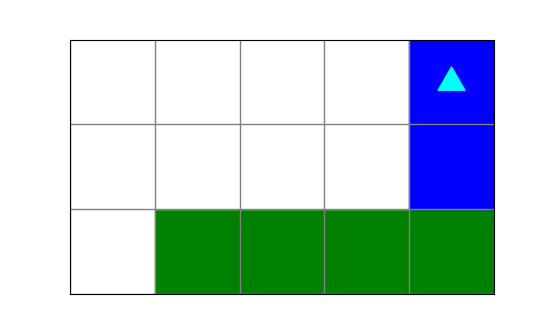b.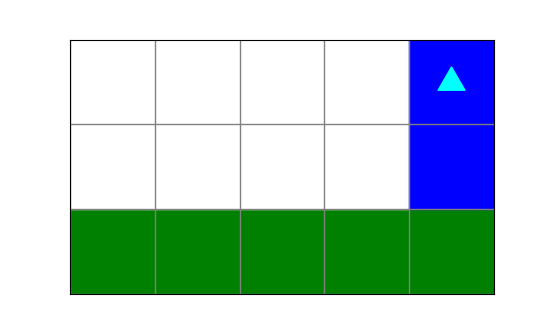c.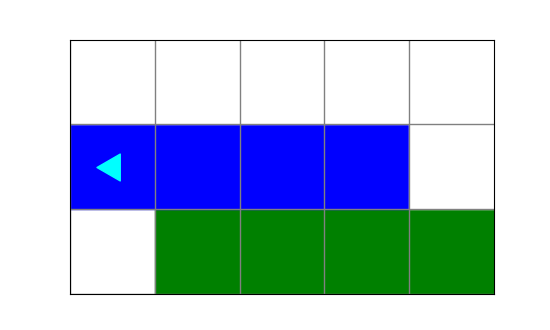d.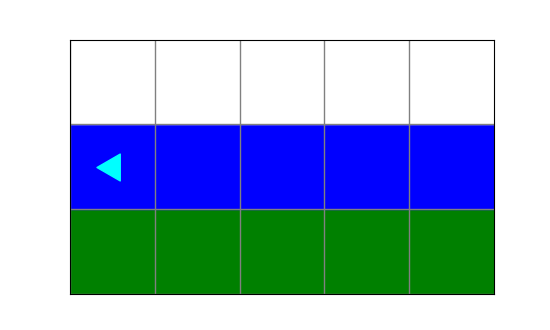### Question 2

You are given this code:

from byubit import Bit

def turn(bit):
bit.left()
bit.move()
bit.left()

def change(bit):
if bit.is_red():
bit.paint('green')
if bit.is_blue():
bit.paint('red')

def do_change(bit):
while bit.front_clear():
bit.move()
change(bit)

And you have this starting world: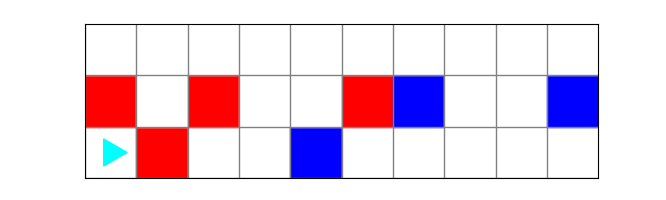and this desired finishing world: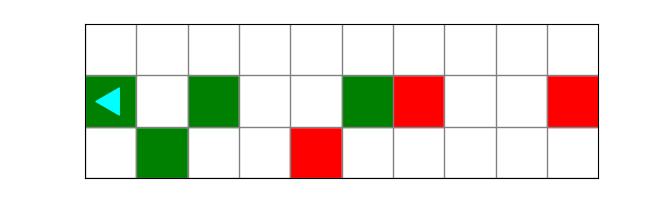Carefully draw out the result of each code block. Which block of code will produce the correct ending world?

a.

@Bit.run('start')
def run(bit):
turn(bit)
do_change(bit)
turn(bit)
do_change(bit)

b.

@Bit.run('start')
def run(bit):
do_change(bit)
turn(bit)
change(bit)
do_change(bit)

c.

@Bit.run('start')
def run(bit):
do_change(bit)
do_change(bit)

d.

@Bit.run('start')
def run(bit):
do_change(bit)
turn(bit)
do_change(bit)

### Question 3

The coyote is stuck and needs to get back on the cliff. You have this starting world: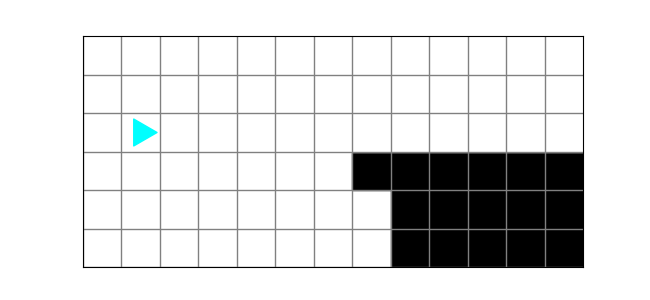and this finishing world: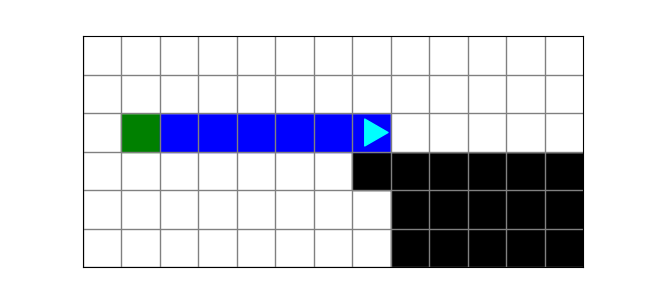Which block of code produces the correct finishing world?

a.

@Bit.run('cliff')
def run(bit):
bit.paint('blue')
while bit.right_clear():
bit.move()
bit.paint('blue')

b.

@Bit.run('cliff')
def run(bit):
bit.paint('green')
while bit.right_clear():
bit.paint('blue')
bit.move()

c.

@Bit.run('cliff')
def run(bit):
bit.paint('green')
while not bit.right_clear():
bit.move()
bit.paint('blue')

d.

@Bit.run('cliff')
def run(bit):
bit.paint('green')
while bit.right_clear():
bit.move()
bit.paint('blue')

### Question 4

What does this code create?

from byubit import Bit

@Bit.run_from_empty(8, 8)
def run(bit):
while not bit.is_blue():
bit.paint('blue')
bit.move()
if not bit.front_clear():
bit.paint('blue')
bit.left()
bit.move()
bit.left()

a.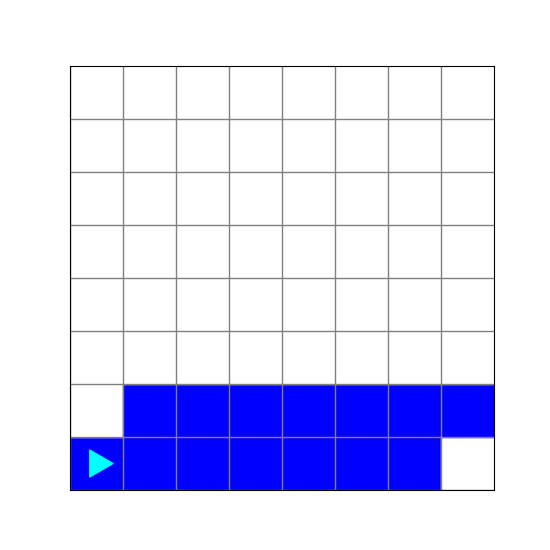b.c.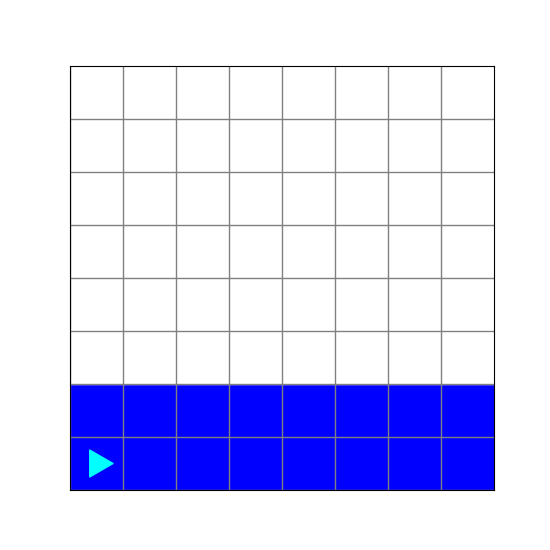d.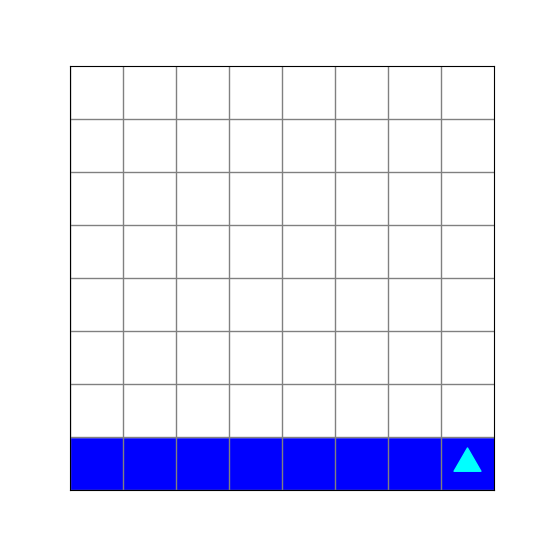## Lists

### Question 5

Which user-defined functions are executed by Python in the following code?

def less(a, b):
if a < b:
return True
else:
return False

def collect(numbers):
new_list = []
current = None
for number in numbers:
if current is None:
new_list.append(number)
current = number
elif less(number, current):
new_list.append(number)
current = number
return new_list

return a + b

some_numbers = [10, 8, 9, 7, 11, 2]
result = collect(some_numbers)
print(result)

a. collect()

b. less()

c. less(), collect()

### Question 6

In the same code above, what is printed?

a. [10, 8, 9, 7, 11, 2]

b. [8, 7, 2]

c. [10, 8, 7, 2]

d. [10, 8, 9, 7, 2]

### Question 7

In the same code above, how many times is less() called?

a. 1

b. 3

c. 5

d. 6

### Question 8

You have a program called average_numbers.py:

import sys

total = int(sys.argv) + int(sys.argv)
average = total / 2
print(average)

If you run this program with the following command:

python average_numbers.py 2 4

what is sys.argv and what is sys.argv?

a. average_numbers.py, 2

b. 2, 4

c. 2, 2

d. 4, 4

### Question 9

What does the following code print?

def average(numbers):
total = 0
count = 0
for number in numbers:
if number > 2 and number < 8:
total += number
count += 1

print(average([1, 10, 5, 3, 8]))

a. 4

b. 2

c. 4.0

d. 2.0

## Strings

### Question 10

What does the following code write in the output file?

def unknown_function2(line):
new_line = ''
for character in line:
if character in 'aeiou':
new_line += '*'
else:
new_line += character

return new_line

def unknown_function1(input_file, output_file):
with open(input_file) as infile:
with open(output_file, 'w') as outfile:
for line in infile:
line = unknown_function2(line)
outfile.write(line)

unknown_function1("input.txt", 'output.txt')

a. The same lines as the input file.

b. Only the lines from the input file that have vowels in them.

c. The same lines as the input file, with vowels replaced with ’*‘.

d. The same lines as the input file, with ‘aeiou’ replaced with ’*.

### Question 11

What does the following code print?


def best(letters):
best_letter = None
for letter in letters:
if letter.isalpha():
if best_letter is None:
best_letter = letter
elif letter == 'a':
best_letter = letter
elif letter == 'b':
best_letter = letter

return best_letter

print(best('xyzababxyz'))

a. x

b. a

c. b

d. z

### Question 12

On average, often will this code print ‘hello’?

import random

count = 100
while count > 0:
choice = random.random()
if choice <= 0.4:
print('hello')
count = count - 1

a. 20 times

b. 30 times

c. 40 times

d. 50 times

### Question 13

What will this code print?

names = ['Adam', 'Bryce', 'Cody', 'Daniel', 'Emmanuel']
scores - [100, 95, 80, 50, 99]

for name, score in zip(names, scores):
if name == 'Daniel':
print(f'The score for {name} is {score}.)

a. The score for Adam is 100.

b. The score for Bryce is 95.

c. The score for Daniel is 50.

d. The score for Daniel is 80.

### Question 14

What does the following code print?

def longest_word(words):
longest = None
for word in words:
if longest is None:
longest = word
if len(word) > len(longest):
longest = word

return longest, len(longest)

print(longest_word(['hello', 'goodbye', 'ten', 'longest']))

a. ‘goodbye’

b. longest

c. (‘goodbye’, 7)

d. (‘longest’, 7)

### Question 15

Consider the following program:

def get_score():
name = input("Name: ")
score = int(input("Score: "))
return name, score

def get_scores():
scores = []
while True:
response = input('\nWhat do you want to do?\n'
if response.upper() == 'Q':
return scores
elif response.upper() == 'A':
scores.append(get_score())
else:
print(f'Unrecognized option: {response}')

def main():
scores = get_scores()
total = 0
for (name, score) in scores:
print(f'{name}, {score}')
total += score
print(f'Average score: {total / len(scores)}')

if __name__ == '__main__':
main()

If you run this program and provide the following input:

A
Jorge
10
A
Emily
8
G
A
Anna
9
q

What does get_score() return the second time it is called?

a. (‘Jorge’, 10)

b. (‘Emily’, 8)

c. (‘Anna’, 9)

d. None